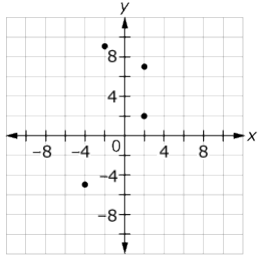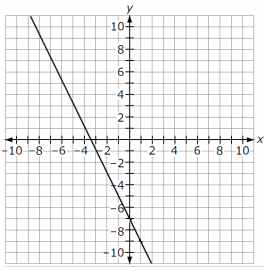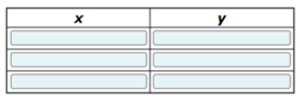# MAFS.8.F.1.1Archived StandardExport Print
Understand that a function is a rule that assigns to each input exactly one output. The graph of a function is the set of ordered pairs consisting of an input and the corresponding output.
General Information
Subject Area: Mathematics
Domain-Subdomain: Functions
Cluster: Level 2: Basic Application of Skills & Concepts
Cluster: Define, evaluate, and compare functions. (Major Cluster) -

Clusters should not be sorted from Major to Supporting and then taught in that order. To do so would strip the coherence of the mathematical ideas and miss the opportunity to enhance the major work of the grade with the supporting clusters.

Date of Last Rating: 02/14
Status: State Board Approved - Archived
Assessed: Yes
Test Item Specifications

• Assessment Limits :
Function notation must not be used. Nonlinear functions may be included for identifying a function.
• Calculator :

Neutral

• Context :

Allowable

Sample Test Items (3)
• Test Item #: Sample Item 1
• Question:

A graph is shown.How do you determine if this is a function or not?

• Difficulty: N/A
• Type: OR: Open Response

• Test Item #: Sample Item 2
• Question:

A graph of a function is shownCreate a table to show the relationship of the values of x to the values of y.• Difficulty: N/A
• Type: TI: Table Item

• Test Item #: Sample Item 3
• Question:

Create a table of values to show a relation that is not a function.

• Difficulty: N/A
• Type: TI: Table Item

## Related Courses

This benchmark is part of these courses.
1205050: M/J Accelerated Mathematics Grade 7 (Specifically in versions: 2014 - 2015, 2015 - 2020, 2020 - 2022, 2022 and beyond (current))
1205070: M/J Grade 8 Pre-Algebra (Specifically in versions: 2014 - 2015, 2015 - 2022, 2022 and beyond (current))
1204000: M/J Foundational Skills in Mathematics 6-8 (Specifically in versions: 2014 - 2015, 2015 - 2022, 2022 and beyond (current))
7812030: Access M/J Grade 8 Pre-Algebra (Specifically in versions: 2014 - 2015, 2015 - 2018, 2018 - 2019, 2019 - 2022, 2022 and beyond (current))
7912115: Fundamental Explorations in Mathematics 2 (Specifically in versions: 2013 - 2015, 2015 - 2017 (course terminated))

## Related Access Points

Alternate version of this benchmark for students with significant cognitive disabilities.

## Related Resources

Vetted resources educators can use to teach the concepts and skills in this benchmark.

## Formative Assessments

Recognizing Functions:

Students are asked to determine whether or not each of two graphs represent functions.

Type: Formative Assessment

Writing Functions:

Students are asked to create their own examples and nonexamples of functions by completing tables and mapping diagrams.

Type: Formative Assessment

What Is a Function?:

Students are asked to define the term function including important properties.

Type: Formative Assessment

Tabulating Functions:

Students are asked to determine whether or not tables of ordered pairs represent functions.

Type: Formative Assessment

Identifying Algebraic Functions:

Students are asked to determine if each of three equations represents a function. Although the task provides equations, in their explanations students can use other representations such as ordered pairs, tables of values or graphs.

Type: Formative Assessment

## Lesson Plans

Select a Healthcare Plan:

Students are asked to determine a procedure for ranking healthcare plans based on their assumptions and the cost of each plan given as a function. Then, they are asked to revise their ranking based on a new set of data.

Type: Lesson Plan

What's My Function?:

Students will determine function rules that have been written on cards taped to their backs. They will suggest input values and peers will provide output values to help them determine their function. They will then graph their functions for additional practice.

Type: Lesson Plan

An Introduction to Functions: How Much Are Playoff Tickets?:

This lesson introduces functions with the real-world example of the cost of tickets for a playoff game. Also, students will determine if tables, graphs, or sets of ordered pairs represent functions or not and explain their reasoning.

Type: Lesson Plan

Linear functions:

In this lesson, students will relate function tables to ordered pairs, find the rule, construct a graph of the data and analyze the date on the graph. They will also be able to do it in reverse order.

Type: Lesson Plan

Functions with Vertical Line Test!:

This is an introductory lesson on functions. Students have the opportunity to review graphing ordered pairs and learning how to use a function table. In addition, students learn the difference between relations and functions and how to distinguish between the two.

Type: Lesson Plan

Function or No Function?:

This resource provides a lesson plan for teaching students how to recognize relations and functions. They will distinguish which relations are also functions and which are not.

Type: Lesson Plan

What Are My Test Scores?:

Students will define what a function is at the beginning of class. Then they will activate prior knowledge by playing "Four Corners". After that there will be an investigation of what a function is and how it relates to the total questions on the assessment and the point value for each question. Then students will analyze different assessments. After that students will present their findings. For homework students will have practice problems. They will also create Cornell Notes from the "What are my Test Scores" activity.

Type: Lesson Plan

## Original Student Tutorial

Driven By Functions:

Learn how to determine if a relationship is a function in this interactive tutorial that shows you inputs, outputs, equations, graphs and verbal descriptions.

Type: Original Student Tutorial

Pennies to Heaven:

The goal of this task is to give students a context to investigate large numbers and measurements. Students need to fluently convert units with very large numbers in order to successfully complete this task. The total number of pennies minted either in a single year or for the last century is phenomenally large and difficult to grasp. One way to assess how large this number is would be to consider how far all of these pennies would reach if we were able to stack them one on top of another: this is another phenomenally large number but just how large may well come as a surprise.

Foxes and Rabbits:

This task emphasizes the importance of the "every input has exactly one output" clause in the definition of a function, which is violated in the table of values of the two populations. Noteworthy is that since the data is a collection of input-output pairs, no verbal description of the function is given, so part of the task is processing what the "rule form" of the proposed functions would look like.

Function Rules:

This task can be played as a game where students have to guess the rule and the instructor gives more and more input output pairs. Giving only three input output pairs might not be enough to clarify the rule. Instructors might consider varying the inputs in, for example, the second table, to provide non-integer entries. A nice variation on the game is to have students who think they found the rule supply input output pairs, and the teachers confirms or denies that they are right. Verbalizing the rule requires precision of language. This task can be used to introduce the idea of a function as a rule that assigns a unique output to every input.

US Garbage, Version 1:

In this task, the rule of the function is more conceptual. We assign to a year (an input) the total amount of garbage produced in that year (the corresponding output). Even if we didn't know the exact amount for a year, it is clear that there will not be two different amounts of garbage produced in the same year. Thus, this makes sense as a "rule" even though there is no algorithmic way to determine the output for a given input except looking it up in the table.

Drip, Drop, Drip, Drop:

Students design an experiment to model a leaky faucet and determine the amount of water wasted due to the leak. Using the data they gather in a table, students graph and write an equation for a line of best fit. Students then use their derived equation to make predictions about the amount of water that would be wasted from one leak over a long period of time or the amount wasted by several leaks during a specific time period.

## Student Center Activity

Students can practice answering mathematics questions on a variety of topics. With an account, students can save their work and send it to their teacher when complete.

Type: Student Center Activity

## Tutorials

Does a Vertical Line Represent a Function?:

This video explains why a vertical line does not represent a function.

Type: Tutorial

Check if a Verbal Description Is a Function:

This video demonstrates how to check if a verbal description represents a function.

Type: Tutorial

How to Check if Points on a Graph Represent a Function:

This video shows how to check whether a given set of points can represent a function. For the set to represent a function, each domain element must have one corresponding range element at most.

Type: Tutorial

Linear Function: Spending Money:

In this tutorial, you will practice using an equation in slope-intercept form to find coordinates, then graph the coordinates to predict an answer to the problem.

Type: Tutorial

Functions:

Functions can be thought of as mathematical machines, which when given an element from a set of permissible inputs, always produce the same element from a set of possible outputs

Type: Tutorial

## Virtual Manipulatives

Functions and Vertical Line Test:

This lesson is designed to introduce students to the vertical line test for functions as well as practice plotting points and drawing simple functions. The lesson provides links to discussions and activities related to the vertical line test and functions as well as suggested ways to integrate them into the lesson.

Type: Virtual Manipulative

Introduction to Functions:

This lesson is designed to introduce students to functions as rules and independent and dependent variables. The lesson provides links to discussions and activities that motivate the idea of a function as a machine as well as proper terminology when discussing functions. Finally, the lesson provides links to follow-up lessons designed for use in succession to the introduction of functions.

Type: Virtual Manipulative

Number Cruncher:

In this activity, students enter inputs into a function machine. Then, by examining the outputs, they must determine what function the machine is performing. This activity allows students to explore functions and what inputs are most useful for determining the function rule. This activity includes supplemental materials, including background information about the topics covered, a description of how to use the application, and exploration questions for use with the java applet.

Type: Virtual Manipulative

Graphs and Functions:

This lesson is designed to introduce students to plotting points and graphing functions in the Cartesian coordinate system. The lesson provides links to discussions and activities that transition from functions as rules to the graphs of those functions. Finally, the lesson provides links to follow-up lessons designed for use in succession to an introduction to graphing.

Type: Virtual Manipulative

## STEM Lessons - Model Eliciting Activity

Select a Healthcare Plan:

Students are asked to determine a procedure for ranking healthcare plans based on their assumptions and the cost of each plan given as a function. Then, they are asked to revise their ranking based on a new set of data.

## MFAS Formative Assessments

Identifying Algebraic Functions:

Students are asked to determine if each of three equations represents a function. Although the task provides equations, in their explanations students can use other representations such as ordered pairs, tables of values or graphs.

Recognizing Functions:

Students are asked to determine whether or not each of two graphs represent functions.

Tabulating Functions:

Students are asked to determine whether or not tables of ordered pairs represent functions.

What Is a Function?:

Students are asked to define the term function including important properties.

Writing Functions:

Students are asked to create their own examples and nonexamples of functions by completing tables and mapping diagrams.

## Original Student Tutorials Mathematics - Grades 6-8

Driven By Functions:

Learn how to determine if a relationship is a function in this interactive tutorial that shows you inputs, outputs, equations, graphs and verbal descriptions.

## Student Resources

Vetted resources students can use to learn the concepts and skills in this benchmark.

## Original Student Tutorial

Driven By Functions:

Learn how to determine if a relationship is a function in this interactive tutorial that shows you inputs, outputs, equations, graphs and verbal descriptions.

Type: Original Student Tutorial

Pennies to Heaven:

The goal of this task is to give students a context to investigate large numbers and measurements. Students need to fluently convert units with very large numbers in order to successfully complete this task. The total number of pennies minted either in a single year or for the last century is phenomenally large and difficult to grasp. One way to assess how large this number is would be to consider how far all of these pennies would reach if we were able to stack them one on top of another: this is another phenomenally large number but just how large may well come as a surprise.

Foxes and Rabbits:

This task emphasizes the importance of the "every input has exactly one output" clause in the definition of a function, which is violated in the table of values of the two populations. Noteworthy is that since the data is a collection of input-output pairs, no verbal description of the function is given, so part of the task is processing what the "rule form" of the proposed functions would look like.

Function Rules:

This task can be played as a game where students have to guess the rule and the instructor gives more and more input output pairs. Giving only three input output pairs might not be enough to clarify the rule. Instructors might consider varying the inputs in, for example, the second table, to provide non-integer entries. A nice variation on the game is to have students who think they found the rule supply input output pairs, and the teachers confirms or denies that they are right. Verbalizing the rule requires precision of language. This task can be used to introduce the idea of a function as a rule that assigns a unique output to every input.

US Garbage, Version 1:

In this task, the rule of the function is more conceptual. We assign to a year (an input) the total amount of garbage produced in that year (the corresponding output). Even if we didn't know the exact amount for a year, it is clear that there will not be two different amounts of garbage produced in the same year. Thus, this makes sense as a "rule" even though there is no algorithmic way to determine the output for a given input except looking it up in the table.

## Student Center Activity

Students can practice answering mathematics questions on a variety of topics. With an account, students can save their work and send it to their teacher when complete.

Type: Student Center Activity

## Tutorials

Does a Vertical Line Represent a Function?:

This video explains why a vertical line does not represent a function.

Type: Tutorial

Check if a Verbal Description Is a Function:

This video demonstrates how to check if a verbal description represents a function.

Type: Tutorial

How to Check if Points on a Graph Represent a Function:

This video shows how to check whether a given set of points can represent a function. For the set to represent a function, each domain element must have one corresponding range element at most.

Type: Tutorial

Linear Function: Spending Money:

In this tutorial, you will practice using an equation in slope-intercept form to find coordinates, then graph the coordinates to predict an answer to the problem.

Type: Tutorial

## Virtual Manipulative

Number Cruncher:

In this activity, students enter inputs into a function machine. Then, by examining the outputs, they must determine what function the machine is performing. This activity allows students to explore functions and what inputs are most useful for determining the function rule. This activity includes supplemental materials, including background information about the topics covered, a description of how to use the application, and exploration questions for use with the java applet.

Type: Virtual Manipulative

## Parent Resources

Vetted resources caregivers can use to help students learn the concepts and skills in this benchmark.

Pennies to Heaven:

The goal of this task is to give students a context to investigate large numbers and measurements. Students need to fluently convert units with very large numbers in order to successfully complete this task. The total number of pennies minted either in a single year or for the last century is phenomenally large and difficult to grasp. One way to assess how large this number is would be to consider how far all of these pennies would reach if we were able to stack them one on top of another: this is another phenomenally large number but just how large may well come as a surprise.

Foxes and Rabbits:

This task emphasizes the importance of the "every input has exactly one output" clause in the definition of a function, which is violated in the table of values of the two populations. Noteworthy is that since the data is a collection of input-output pairs, no verbal description of the function is given, so part of the task is processing what the "rule form" of the proposed functions would look like.